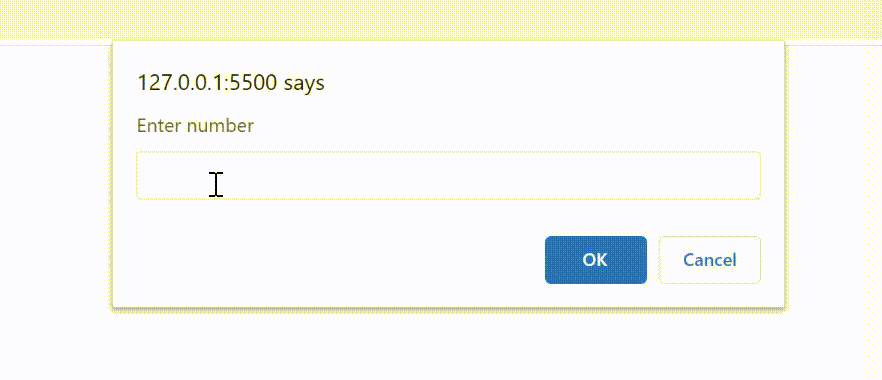Related Articles

# How to create dynamic length array with numbers and sum the numbers using JavaScript ?

• Last Updated : 05 Nov, 2020

In JavaScript, array is a single variable that is used to store different elements. It is often used when we want to store list of elements and access them by a single variable. Unlike most languages where array is a reference to the multiple variable, in JavaScript array is a single variable that stores multiple elements.

JavaScript Arrays are Dynamic in nature it means, the length of array can be modified at run time (when necessary). Array length can be specified after declaring array as shown in the following example.

Example:

## Javascript

 `// Declare an array of undefined size``var` `arr = [];`` ` `// Set array length to 10``arr.length = 10;`` ` `// Print the length to console``console.log(arr);`

Output:

```Array(10) [ <10 empty slots> ]
```

As you can see, this method explain the array with 10 empty slots. The following example declares an array and take input elements using prompt dynamically and print the sum of all elements and display the result sing alert.

Example:

## Javascript

 `(``function` `() {``     ` `    ``// Declare an array of udefined size``    ``var` `arr = [];`` ` `    ``// Notice how the size of array``    ``// is not dot defined`` ` `    ``// Initiating loop to iterate``    ``// for inputs``    ``for` `(``var` `i = 0; i < 10; i++) { `` ` `        ``// Feeding integer inputs to array``        ``arr[i] = +prompt(``'Enter number'``);``         ` `        ``// Calculate sum of array elements``        ``var` `sum = arr.reduce(``function` `(a, b) {``            ``return` `a + b;``        ``}, 0)``    ``}``    ``alert(sum);``})();`

Output:

```Input : 1 2 3 4 5 6 7 8 9 10
Output :55```My Personal Notes arrow_drop_up